# Estimating the area by throwing random points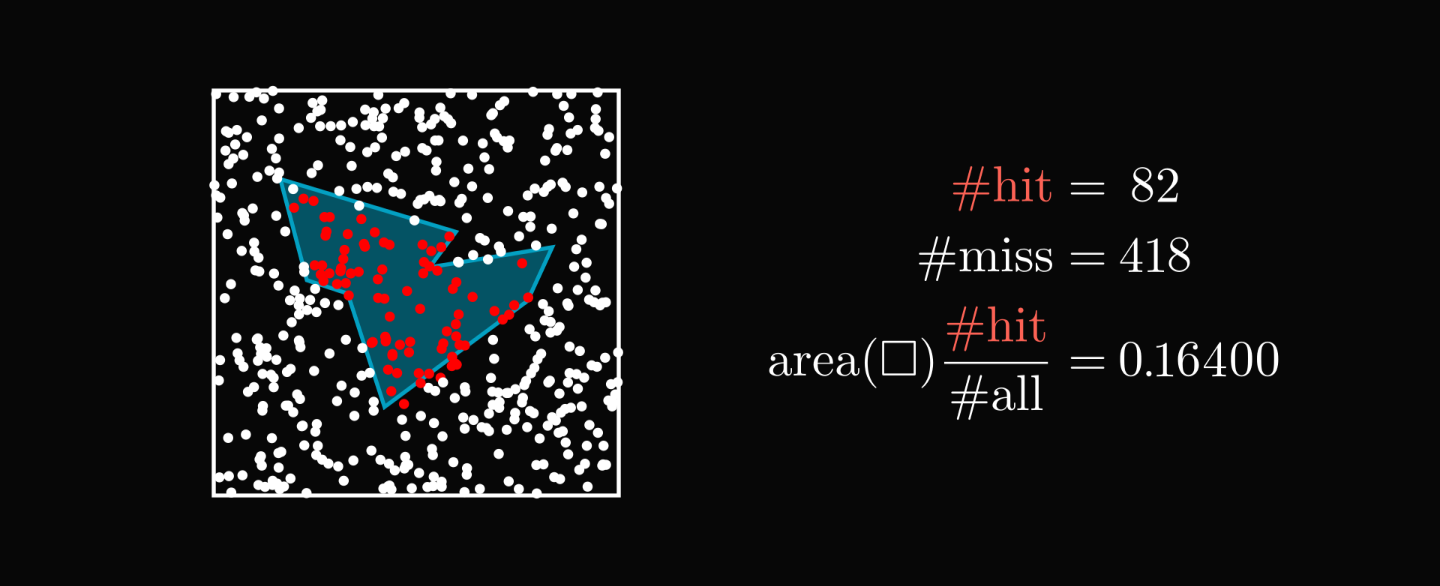One of the coolest ideas in mathematics is the estimation of a shape's area by throwing random points at it.

Don't believe this works? Check out the animation below, where I show the method on the unit circle. (Whose area equals to $\pi$.)

Here is what's behind the magic.

Let's make this method precise! The first step is to enclose our shape $S$ in a square. You can imagine this as a rectangular dartboard.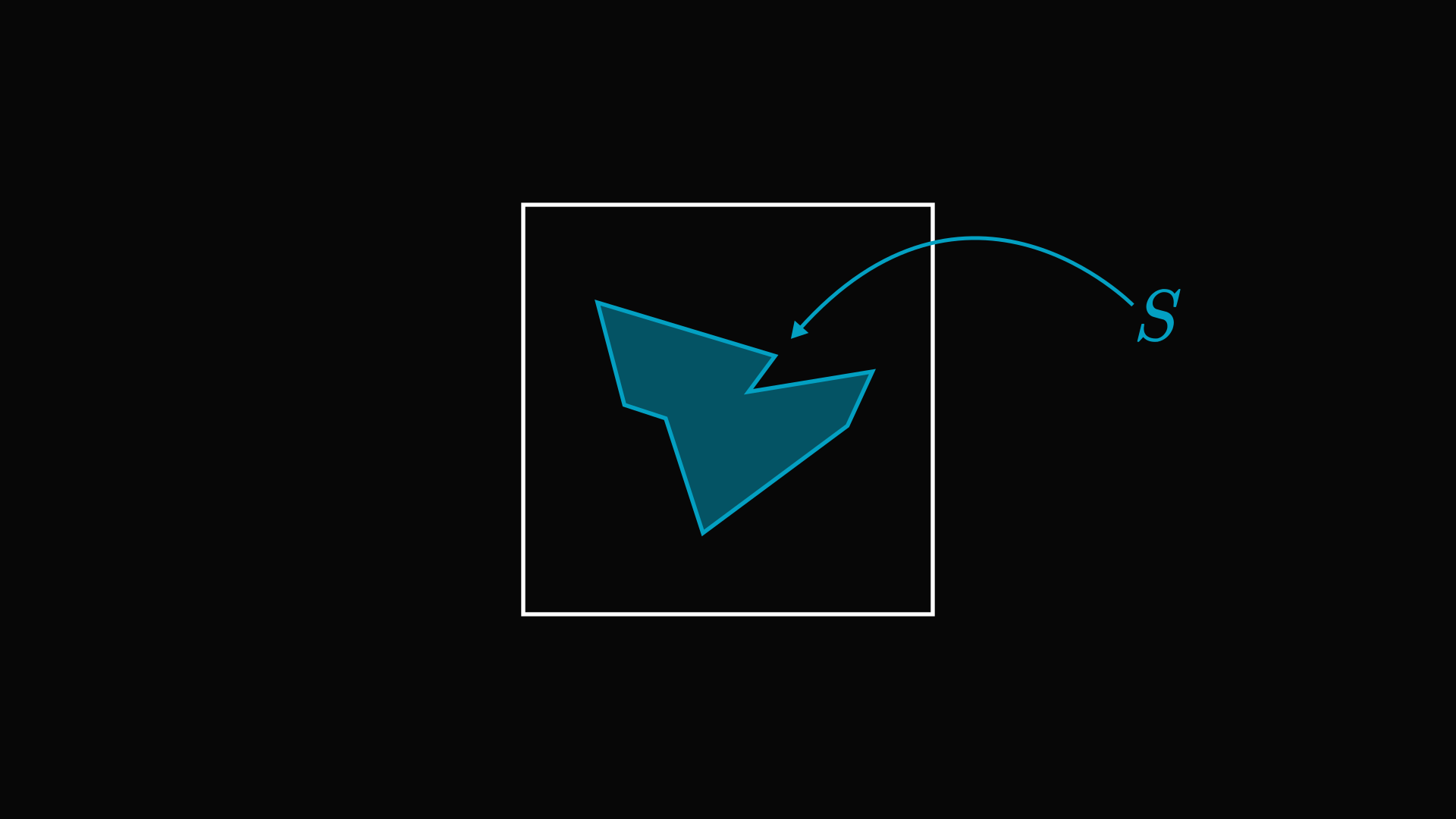Now, we select random points from the board and count how many hit the target.

Again, you can imagine this as closing your eyes, doing a 360° spin, then launching a dart. (Suppose that you always hit the board. Yes, I know. But in math, reality doesn't limit imagination.)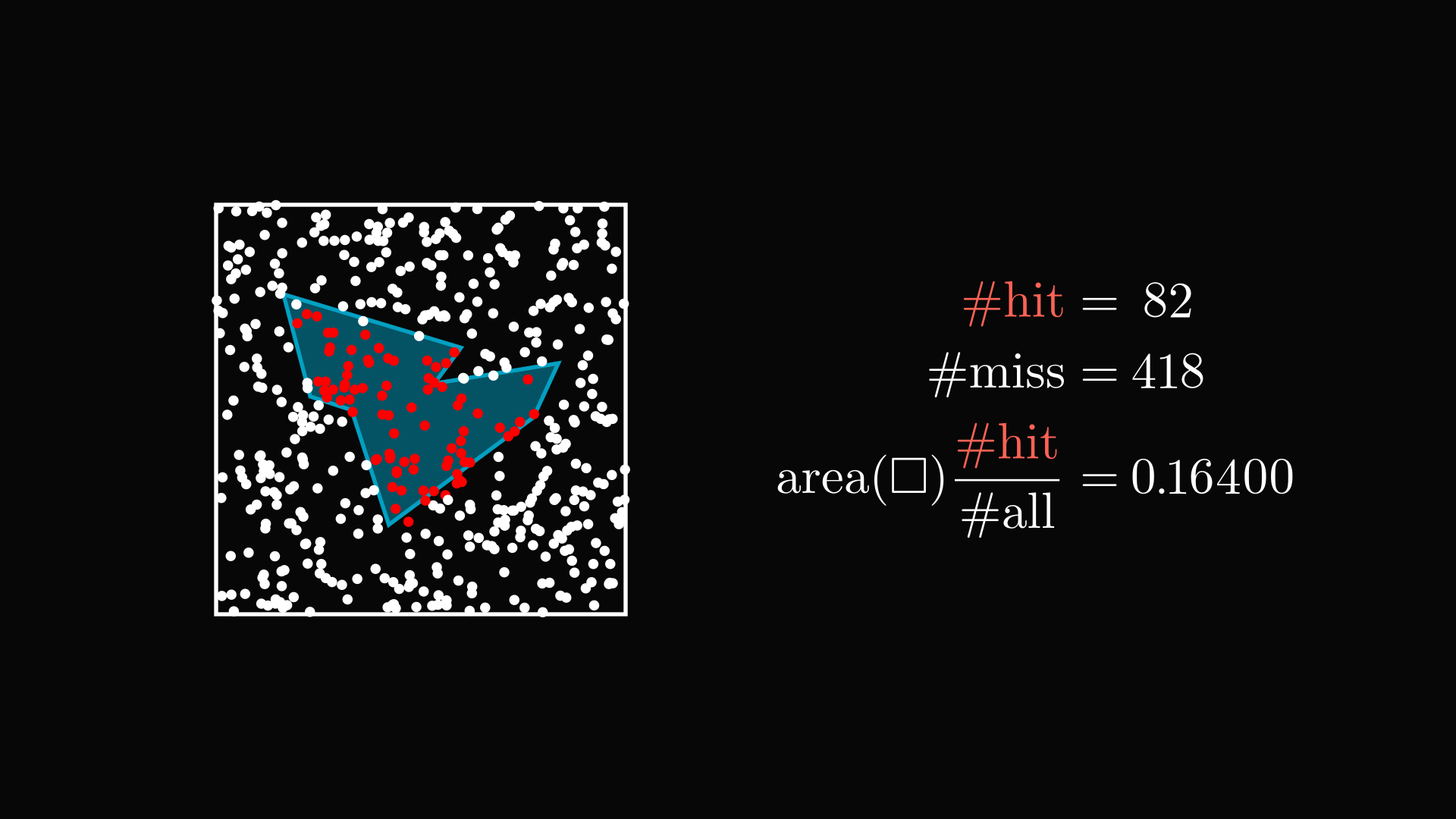The proportion of points inside the shape will approximate the ratio of areas.

Why on earth does this work?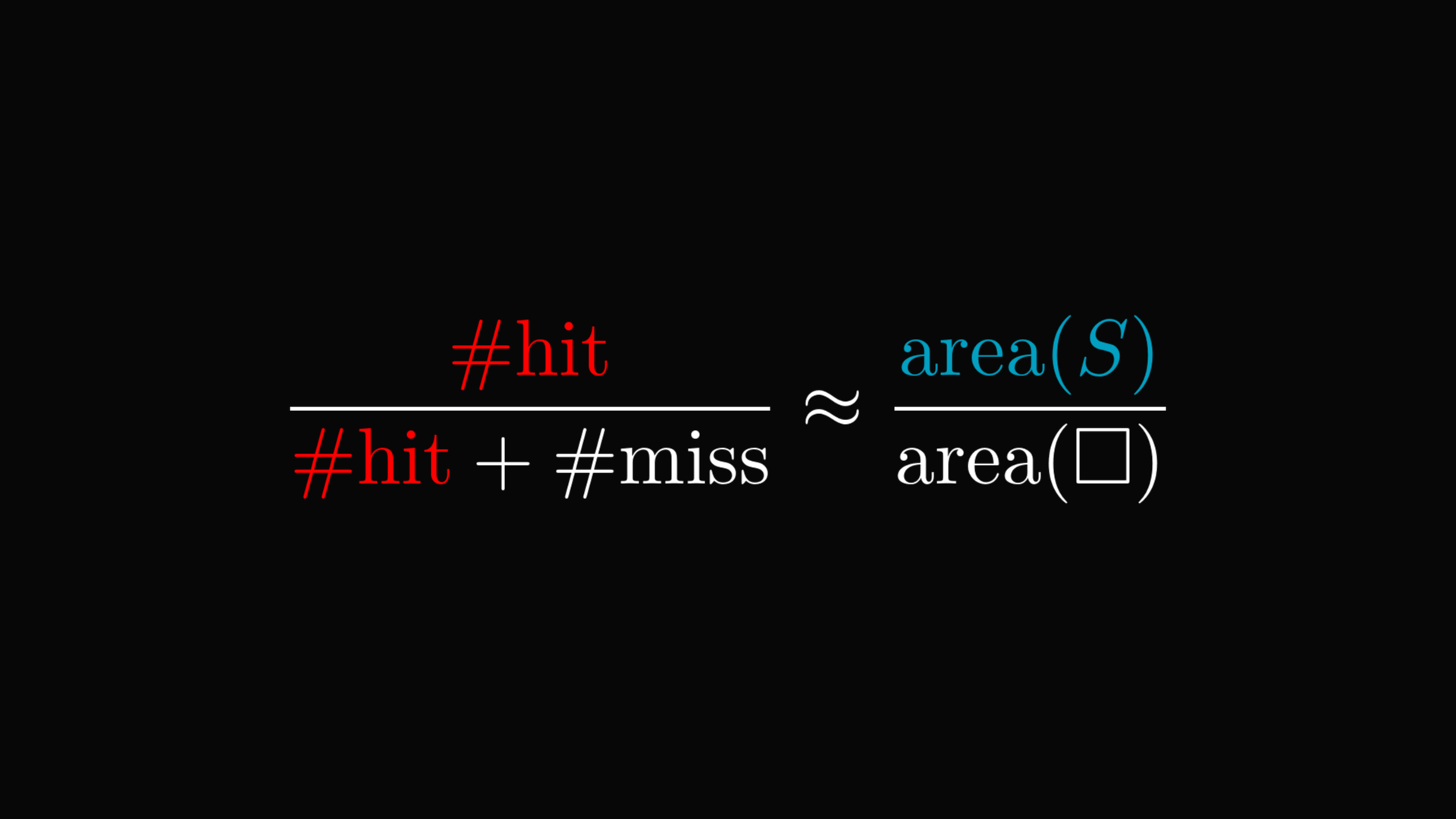Let's start with the definition of "random". There are many ways of selecting a random point from the board.

We use the uniform distribution. This means that the probability of the point hitting our shape is the ratio of the areas.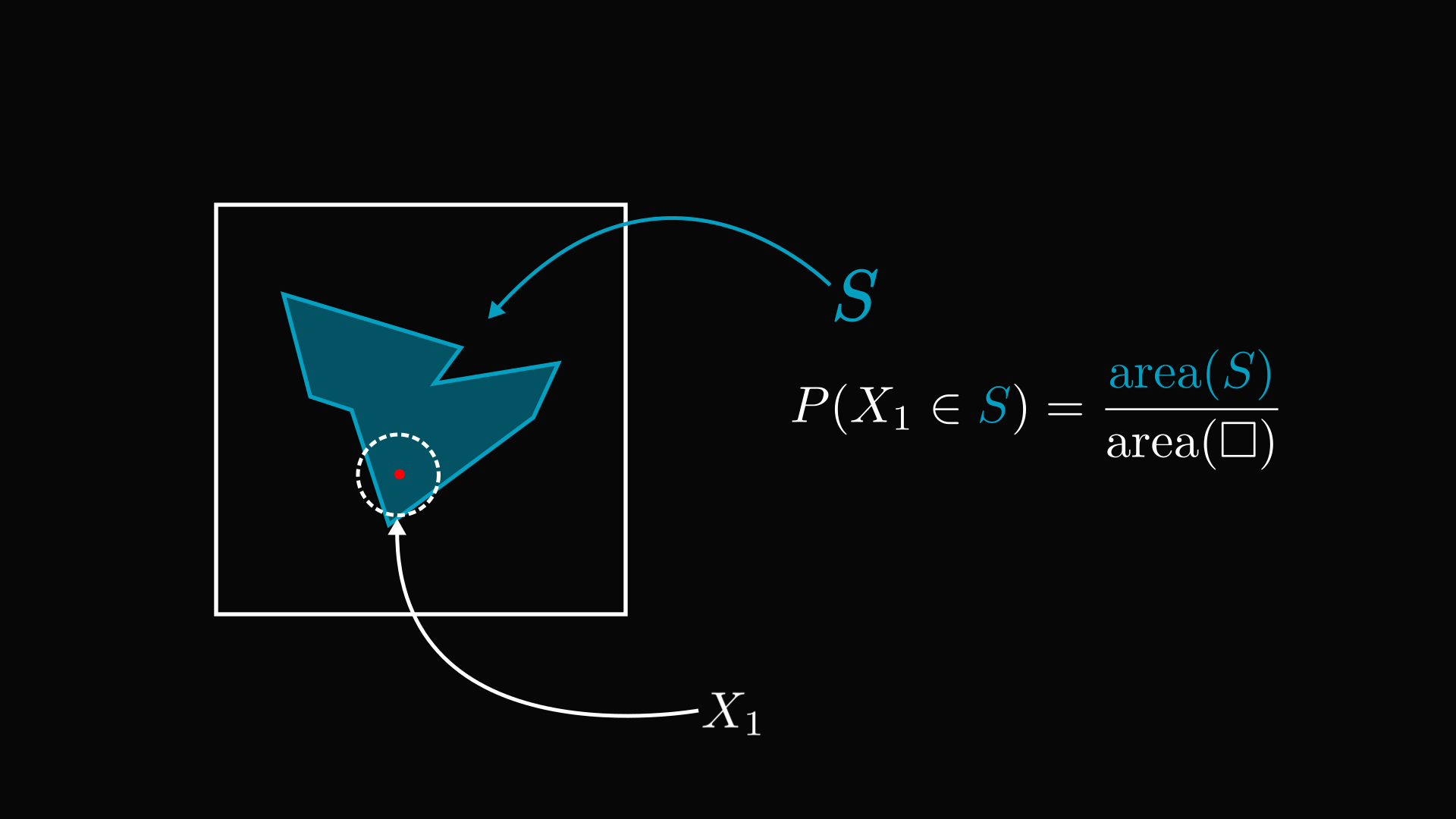We don't care about the exact position of a random point, just whether it hits our shape or not.

Thus, we describe each "dart" by a $0-1$ value. $0$ if it is a miss, $1$ if it is a hit.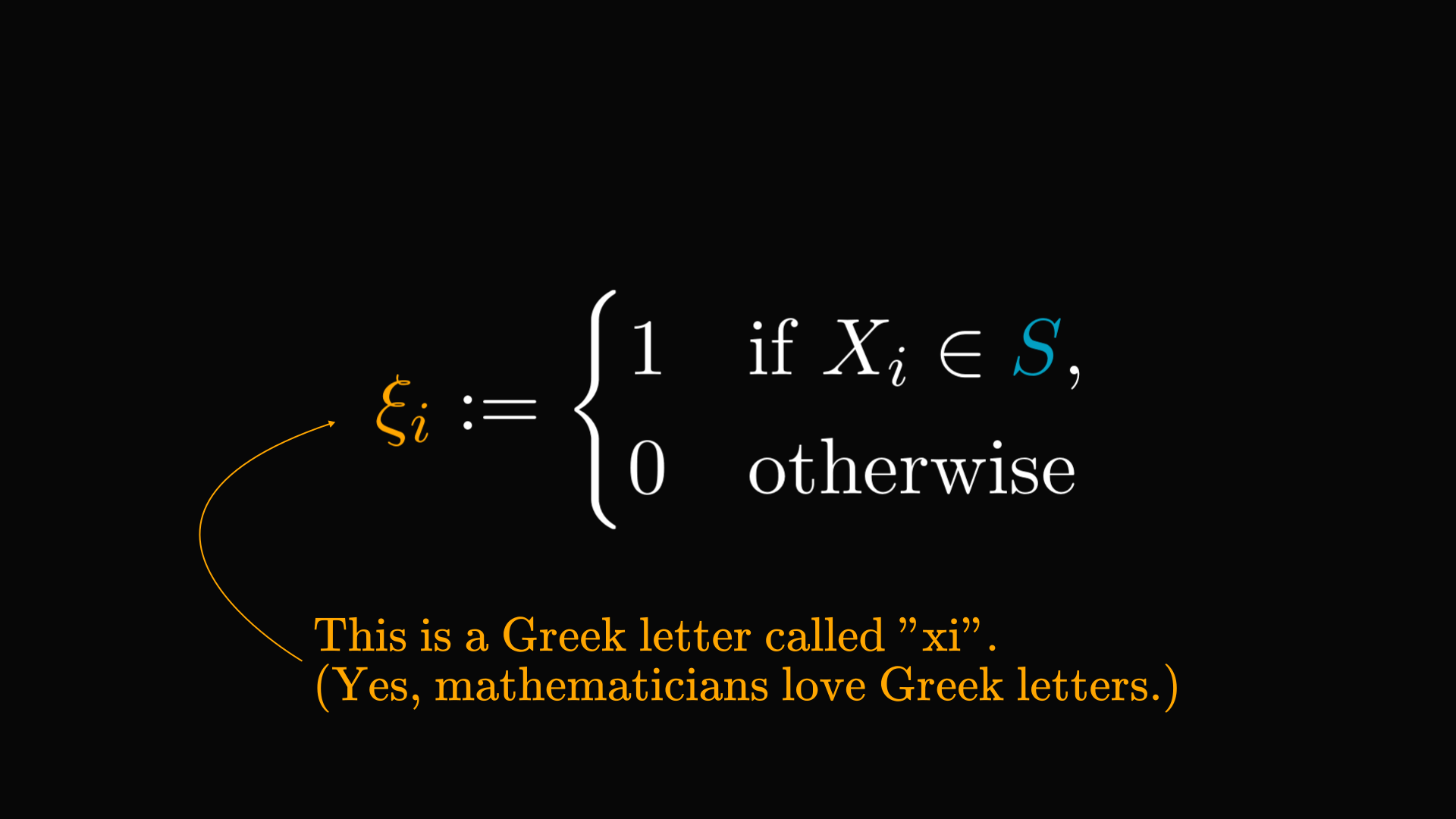In the language of probability, the $\xi$-s are called Bernoulli random variables. Each random point is independent of the others, and their distribution is identical.

Thus, the Law of Large Numbers holds: their average (almost surely) converges to the expected value. (Our Bernoulli variables are identically distributed, so their expected values are equal.)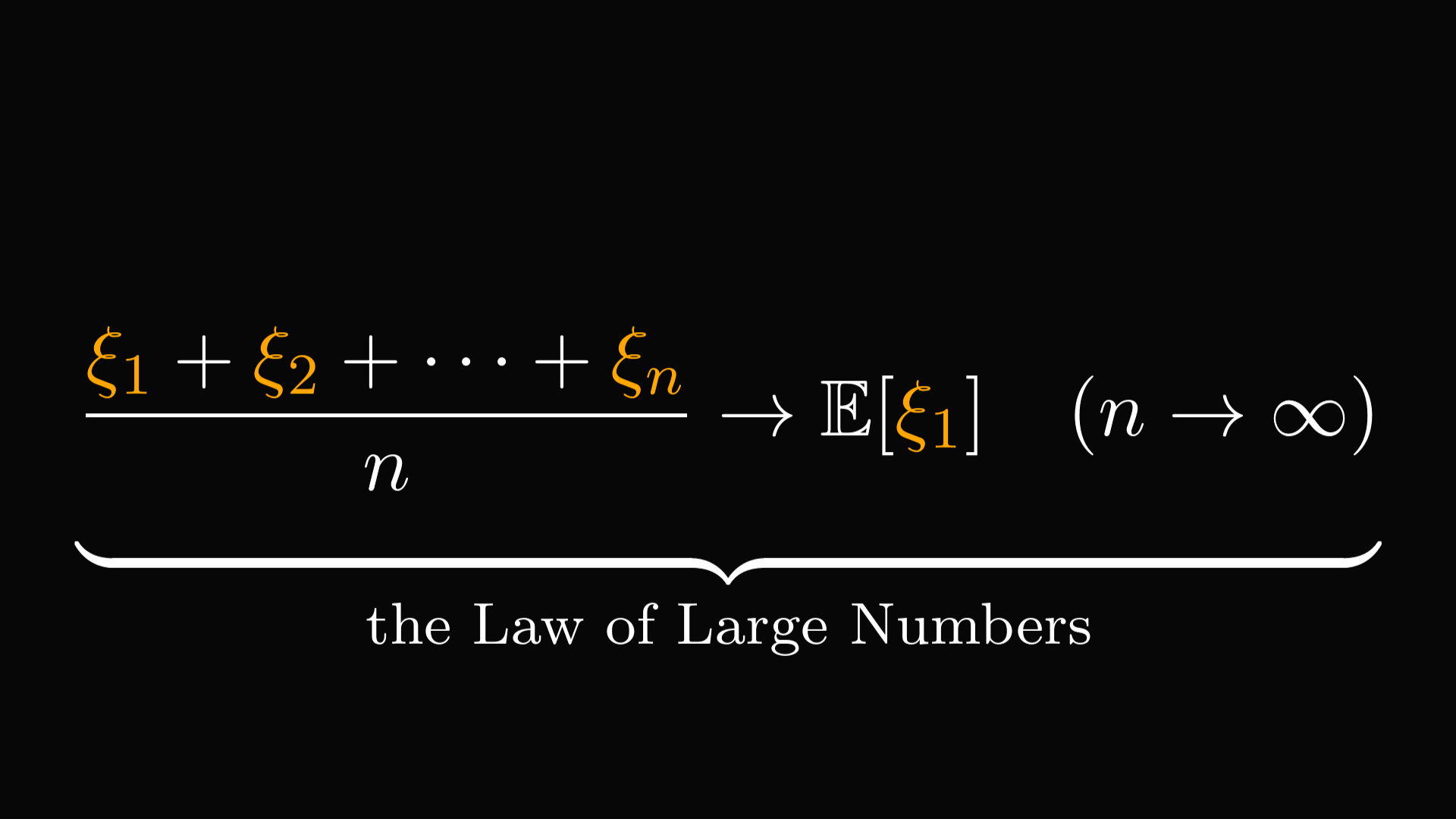On a second look, the average can be written as the total number of hits divided by the total number of points. (Recall that the value of each variable is $0$ if it is a miss and $1$ if it is a hit.)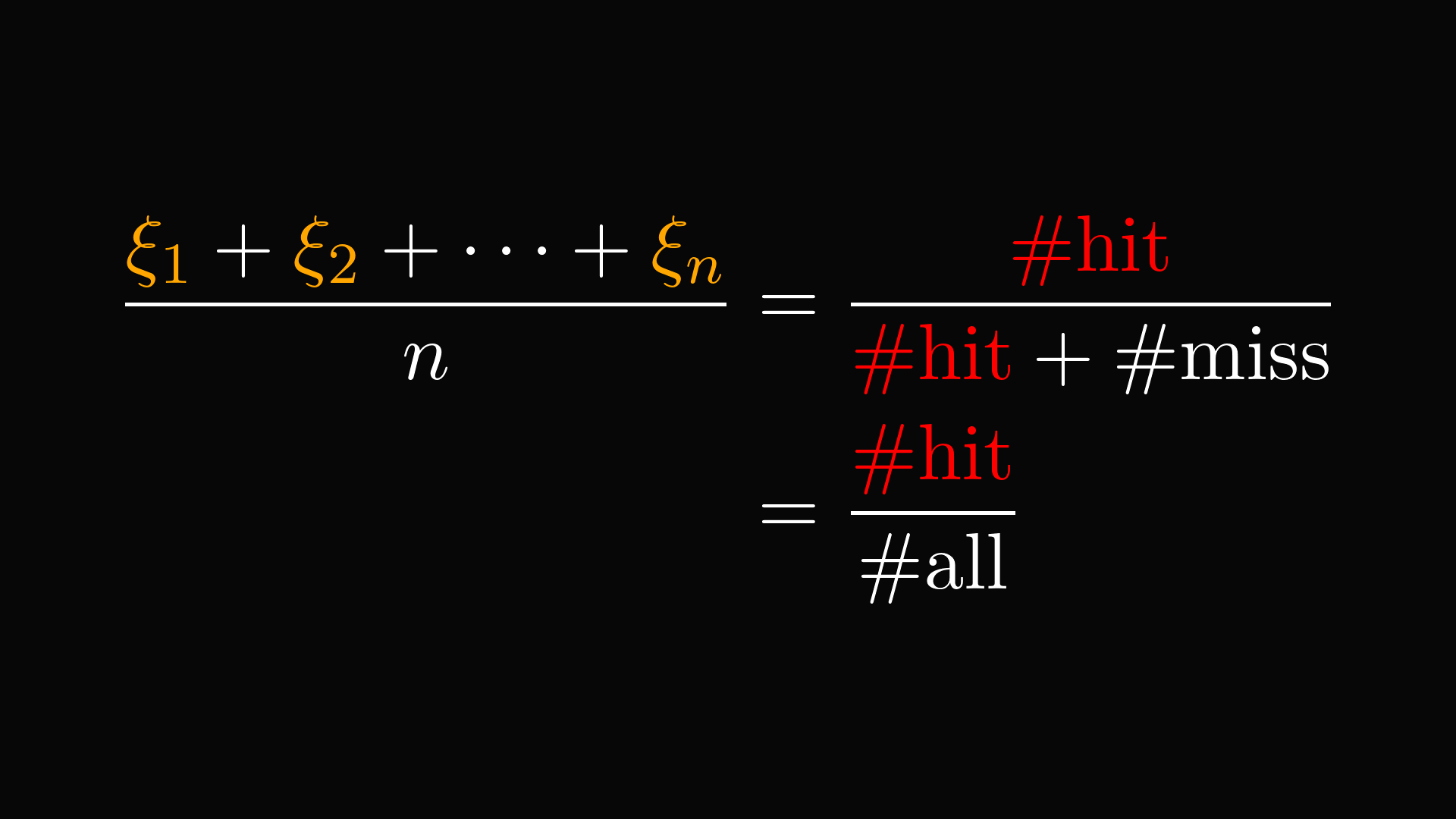On the other hand, the expected value is the probability of a hit. That is, the area of our shape divided by the area of the rectangular board!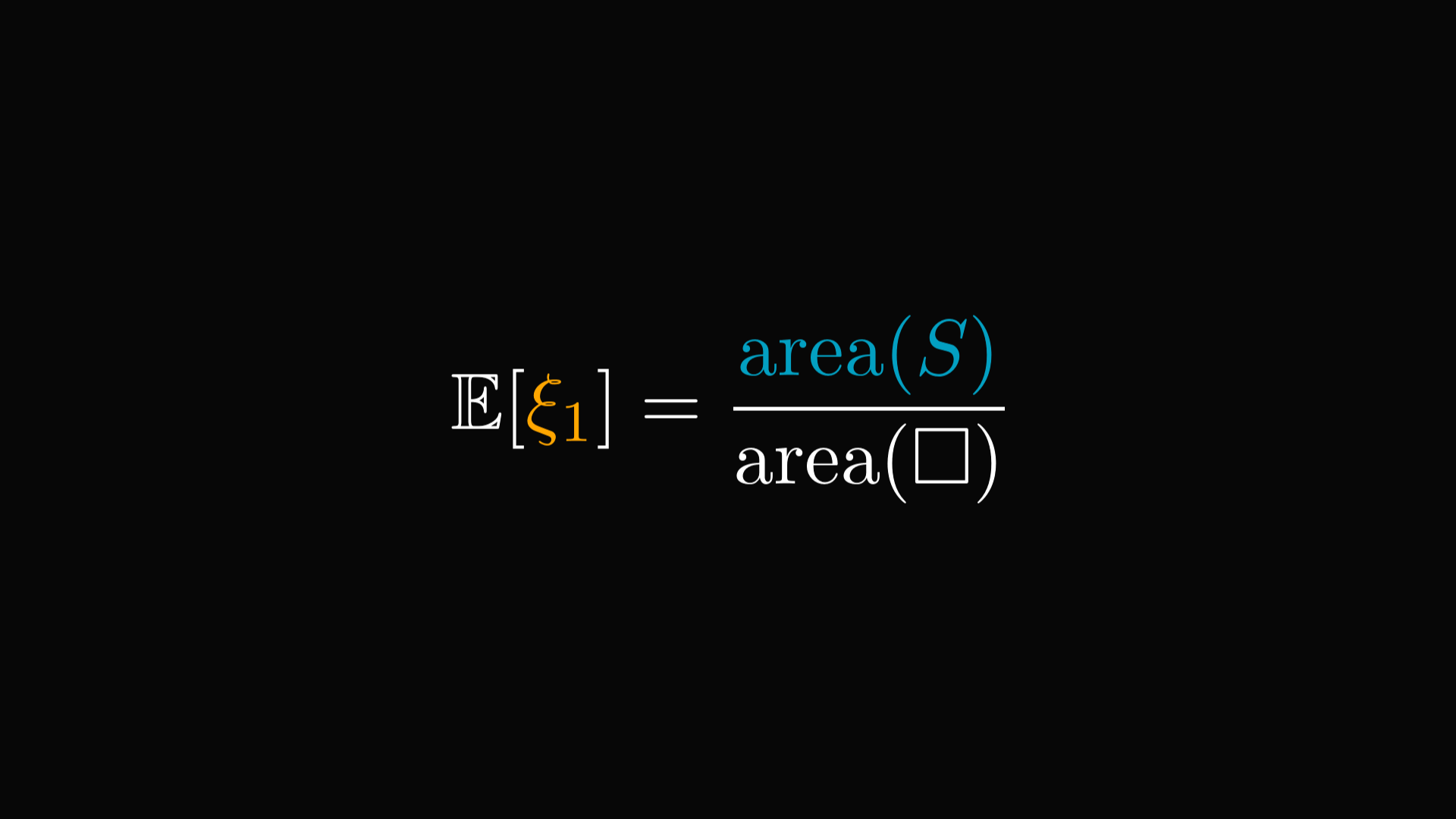Combining these two observations, we get that the frequency of hits converges to the ratio of the areas.

Thus, we can approximate the area by simply counting the number of hits.

This is one of the coolest ideas in mathematics.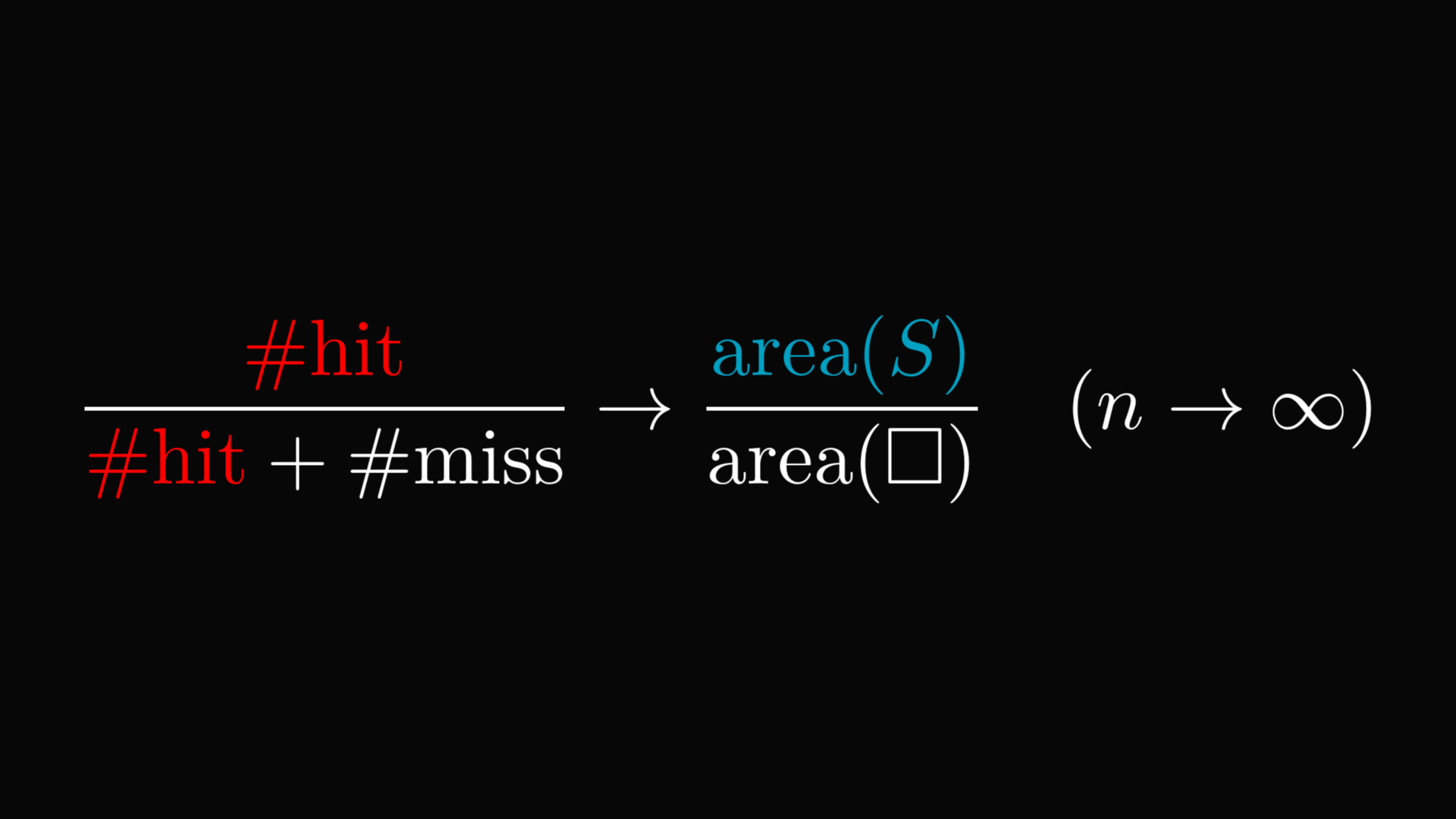The general method is called "Monte Carlo integration", and as the name suggests, it can be used to evaluate integrals of chunky functions. Even ones with lots of variables.

There are drawbacks, like the slow convergence, which has a rate of $1/\sqrt{n}$, where n is the number of points selected.

However, there is no denying it: estimating the area of an object by throwing random points is pretty awesome.